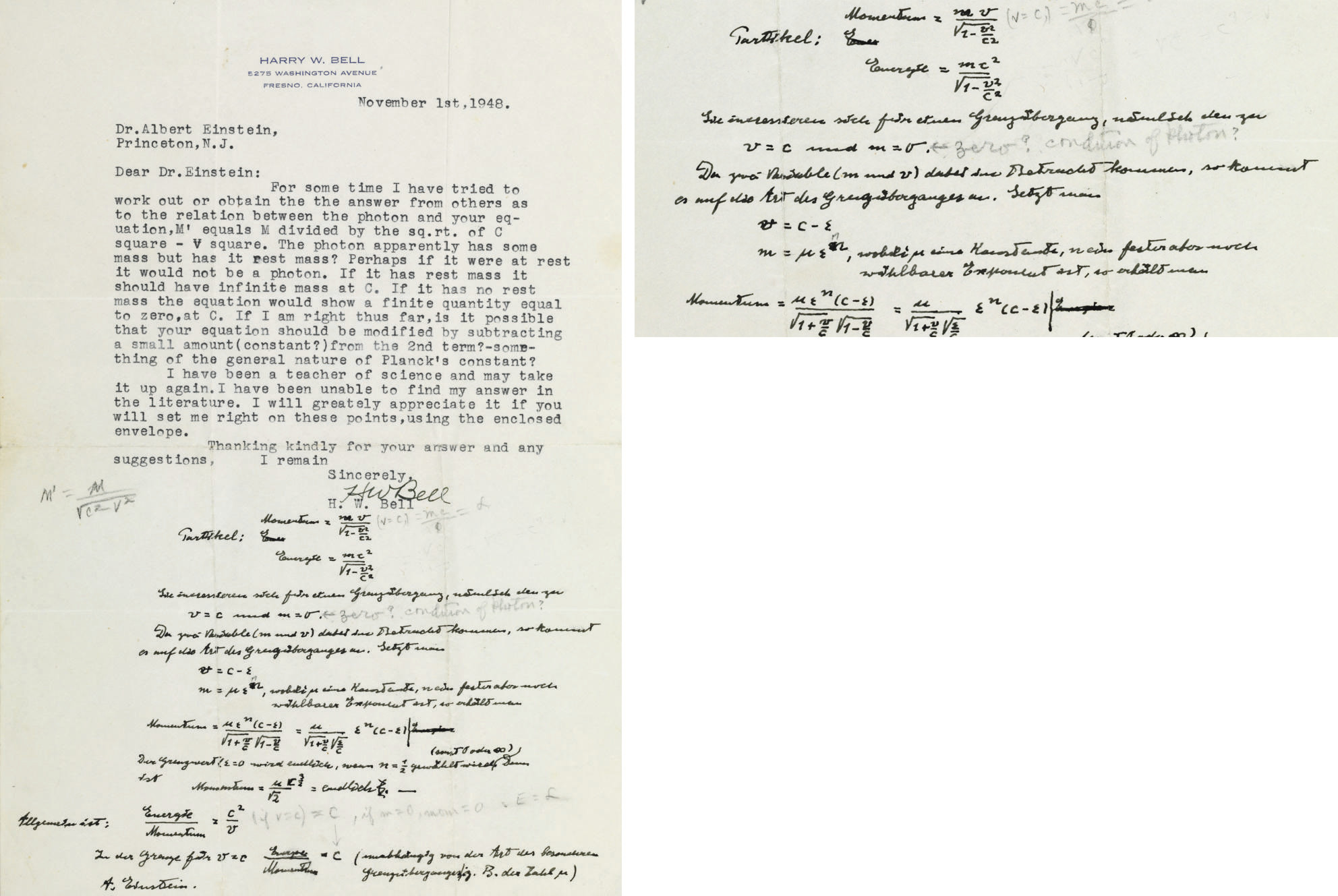248PROPERTY FROM A PRIVATE COLLECTOR
EINSTEIN, Albert (1879-1955). Autograph scientific manuscript signed ("A. Einstein"), INCLUDING HIS FAMOUS FORMULA "ENERGY=MC2...", added in blank portion of a Typed letter signed from H. W. Bell to Einstein, 1 November 1948. 1 page, 4to, with pencil notations in another hand. Einstein's text in German.
Details
EINSTEIN, Albert (1879-1955). Autograph scientific manuscript signed ("A. Einstein"), INCLUDING HIS FAMOUS FORMULA "ENERGY=MC2...", added in blank portion of a Typed letter signed from H. W. Bell to Einstein, 1 November 1948. 1 page, 4to, with pencil notations in another hand. Einstein's text in German.

E = MC2...EINSTEIN EXPLAINS HIS RELATIVITY THEORY USING HIS FAMOUS EQUATION

Einstein answers a query from H. W. Bell, a former "teacher of science." Bell asks about "the relation between the photon and your equation...The photon apparently has some mass but is it rest mass? Perhaps if it were at rest it would not be a photon. If it has rest mass it should have infinite mass at C..." In response, Einstein writes out a series of complex equations, starting with "Momentum = mv the square root of 2-v2/c2," then his seminal: "Energie = mc2 square root of 1-v2/c2." Einstein's extensive notations comprise ca.60 words and some 6 separate equations in dark, very legible ink. He signs "A. Einstein" at bottom left. Scientists at the Einstein Papers project advise that the equations represent Einstein's elucidation of a basic tenet of the special theory of relativity: "Essentially he shows Bell how to understand the continuity between the two cases [Bell] posits, that of a zero rest mass particle moving at the speed of light, and a very low rest mass particle (not quite zero) moving at nearly the speed of light." Unpublished.

An exceptional example of Einstein's generosity in responding to inquiries from previously unknown correspondents.

View All